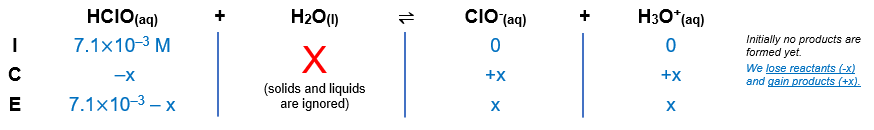# Problem: The acid-dissociation constant for hypochlorous acid (HClO) is 3.0x10 - 8.Calculate the concentration of HClO at equilibrium if the initial concentration of HClO is 7.1×10−3 M.

###### FREE Expert Solution

Equilibrium reaction:        HClO(aq) + H2O(l)  ClO-(aq) + H3O+(aq)

Step 1: Construct an ICE chart for the reaction.Step 2: Write the Ka expression

${\mathbf{K}}_{{\mathbf{a}}}\mathbf{=}\frac{\mathbf{products}}{\mathbf{reactants}}\phantom{\rule{0ex}{0ex}}{\mathbf{K}}_{\mathbf{a}}\mathbf{=}\frac{\mathbf{\left[}{\mathbf{ClO}}^{\mathbf{-}}\mathbf{\right]}\mathbf{\left[}{\mathbf{H}}_{\mathbf{3}}{\mathbf{O}}^{\mathbf{+}}\mathbf{\right]}}{\left[\mathrm{HClO}\right]}$

Solids and liquids are not included in the expression

Step 3: Calculate for x.

81% (390 ratings)###### Problem Details

The acid-dissociation constant for hypochlorous acid (HClO) is 3.0x10 - 8.

Calculate the concentration of HClO at equilibrium if the initial concentration of HClO is 7.1×10−3 M.